Univerza na Primorskem Fakulteta za matematiko, naravoslovje in informacijske tehnologije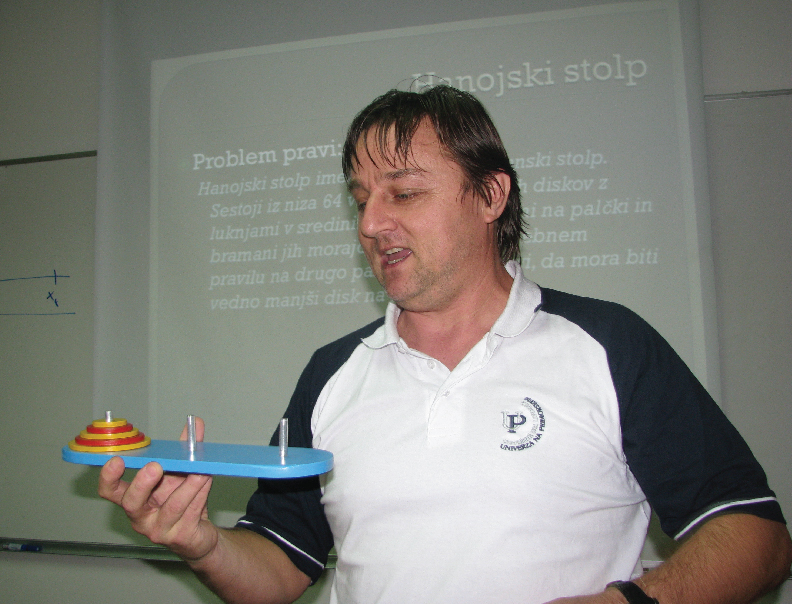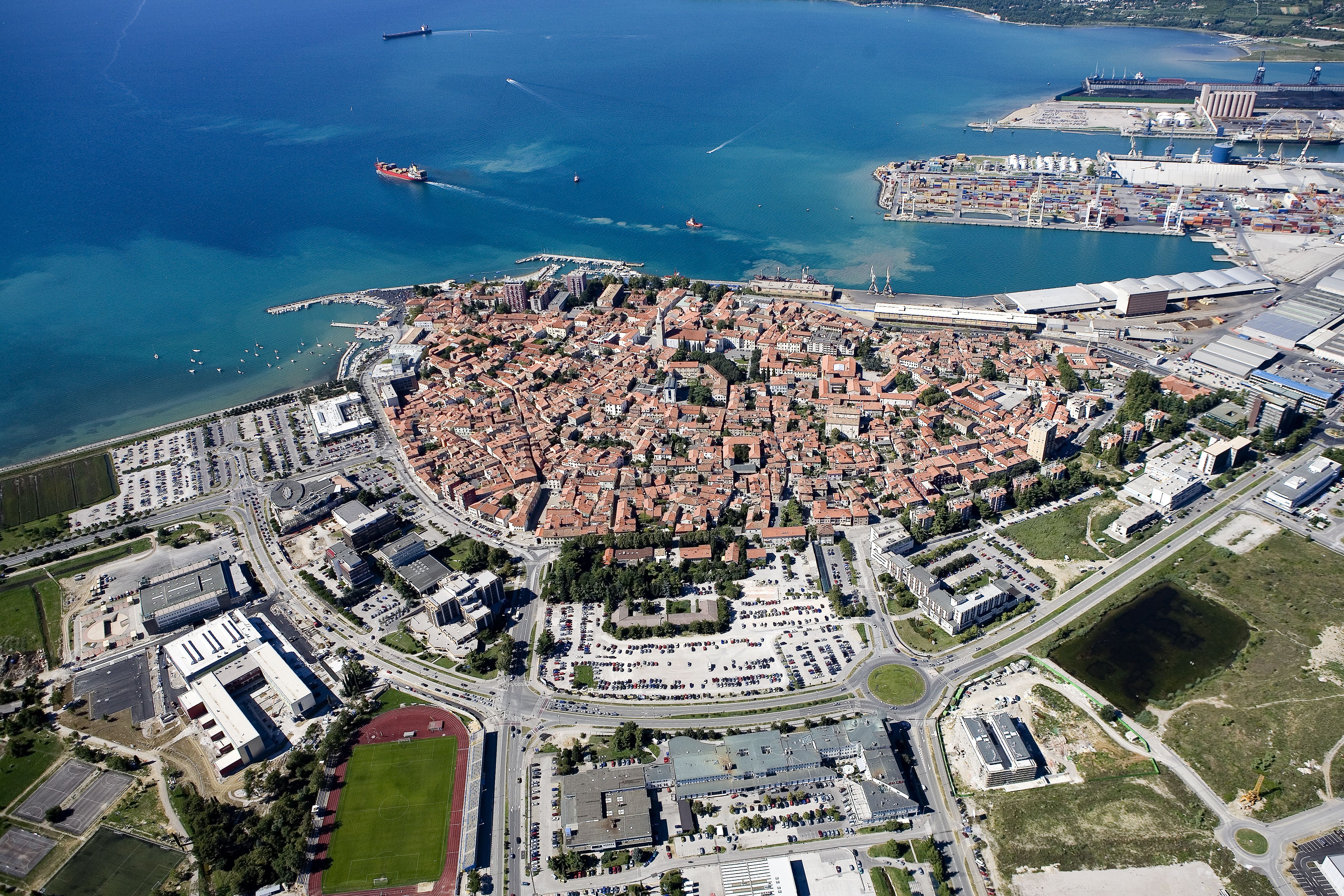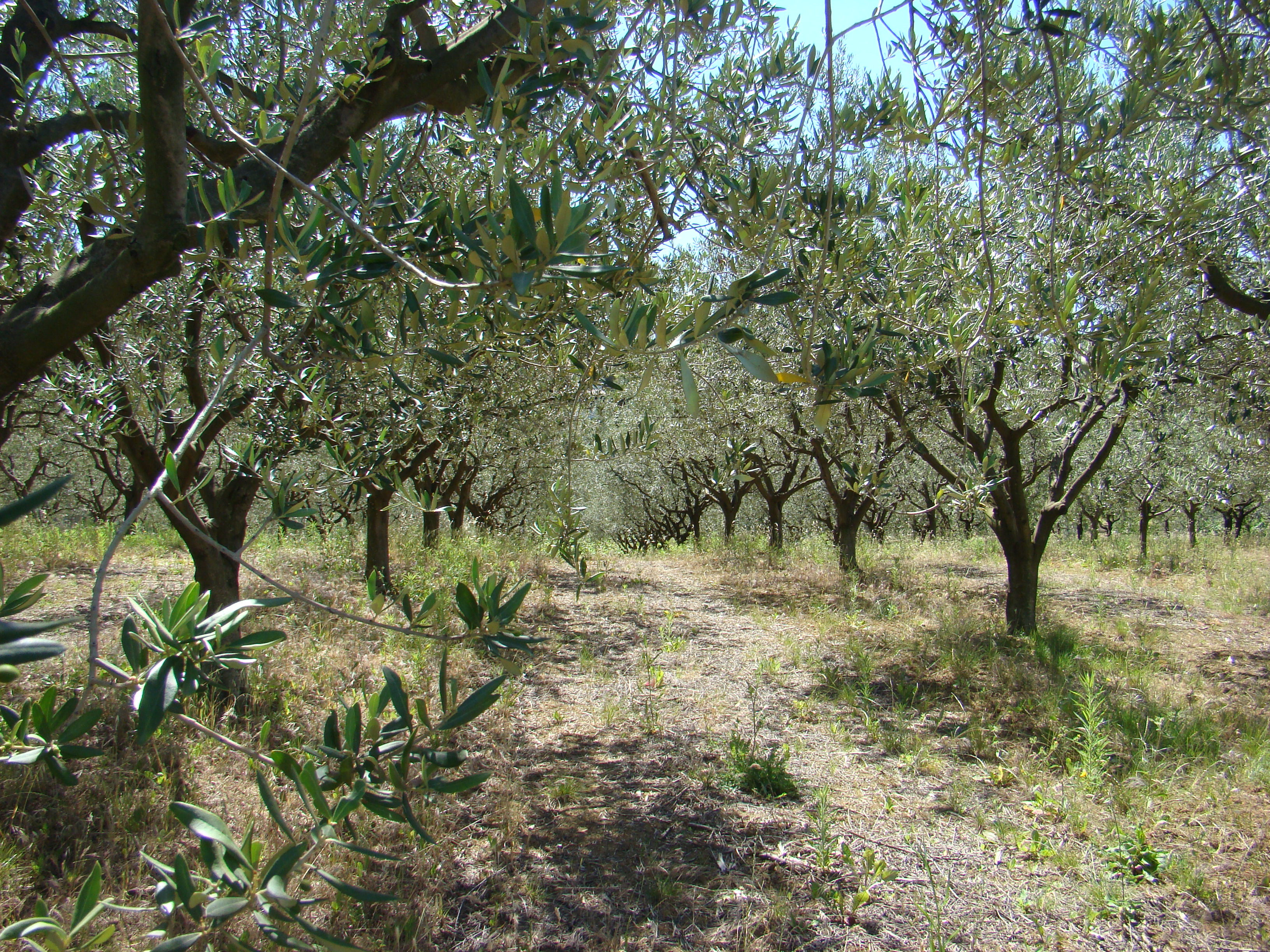SI | EN# Raziskovalni matematični seminar - Arhiv

 2021 2020 2019 2018 2017 2016 2015 2014 2013 2012 2011 2010
 1 2 3 4 5 6 7 8 9 10 11 12
Datum in ura / Date and time: 29.5.17
(10:00-11:00)
Predavalnica / Location: FAMNIT-POŠTA
Predavatelj / Lecturer: Janko Gravner (University of California, Davis, USA)
Naslov / Title: Jigsaw percolation
Vsebina / Abstract:

Jigsaw percolation is a model for collaborative problem solving: a nonlocal process that iteratively merges connected clusters in a puzzle graph by using connectivity properties of people graph on the same set of vertices. We presume the people graph is random while the puzzle graph is a fixed deterministic graph. The main question is to estimate the probability that the puzzle is solved, that is, that the process eventually produces a single cluster. Particularly sharp answers can be obtained for the one dimensional ring puzzle.

The talk is on joint work with David Sivakoff.

Presentation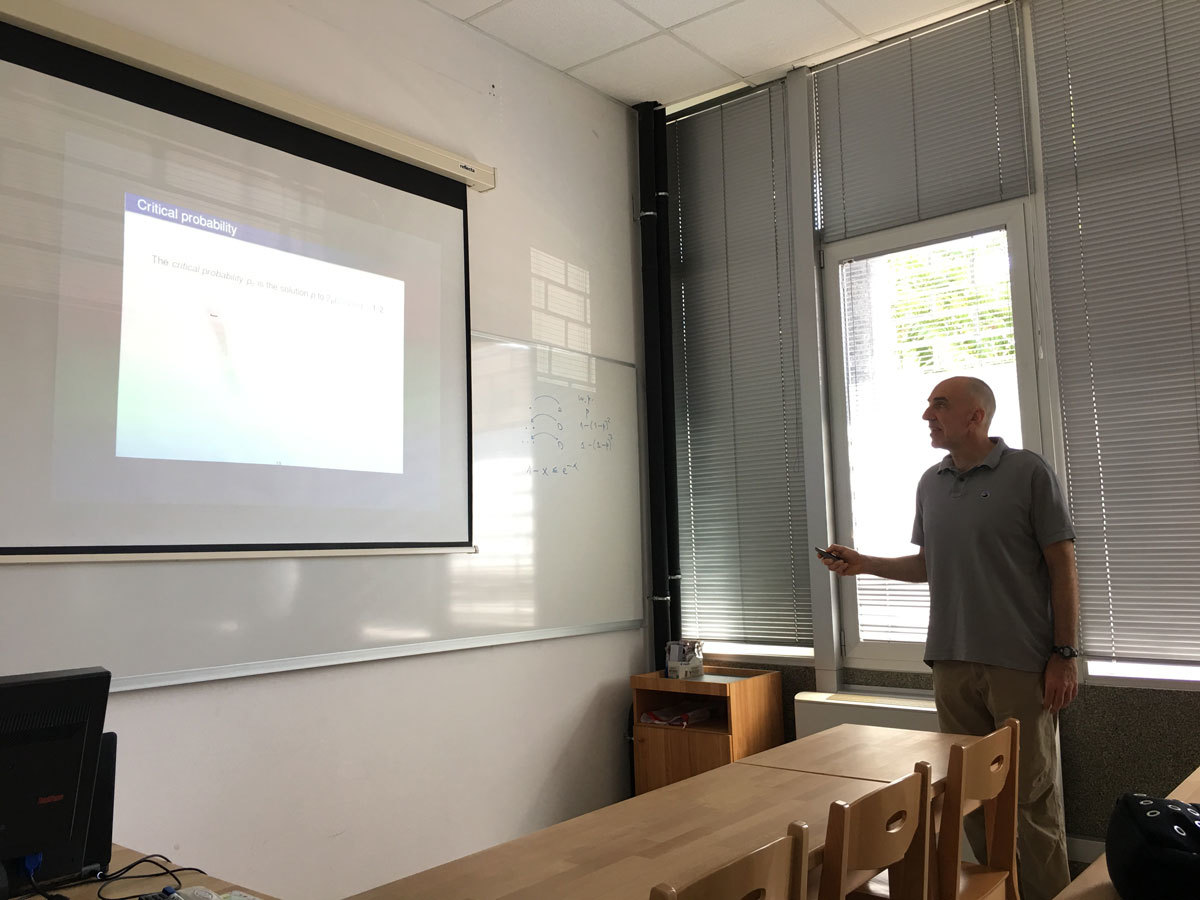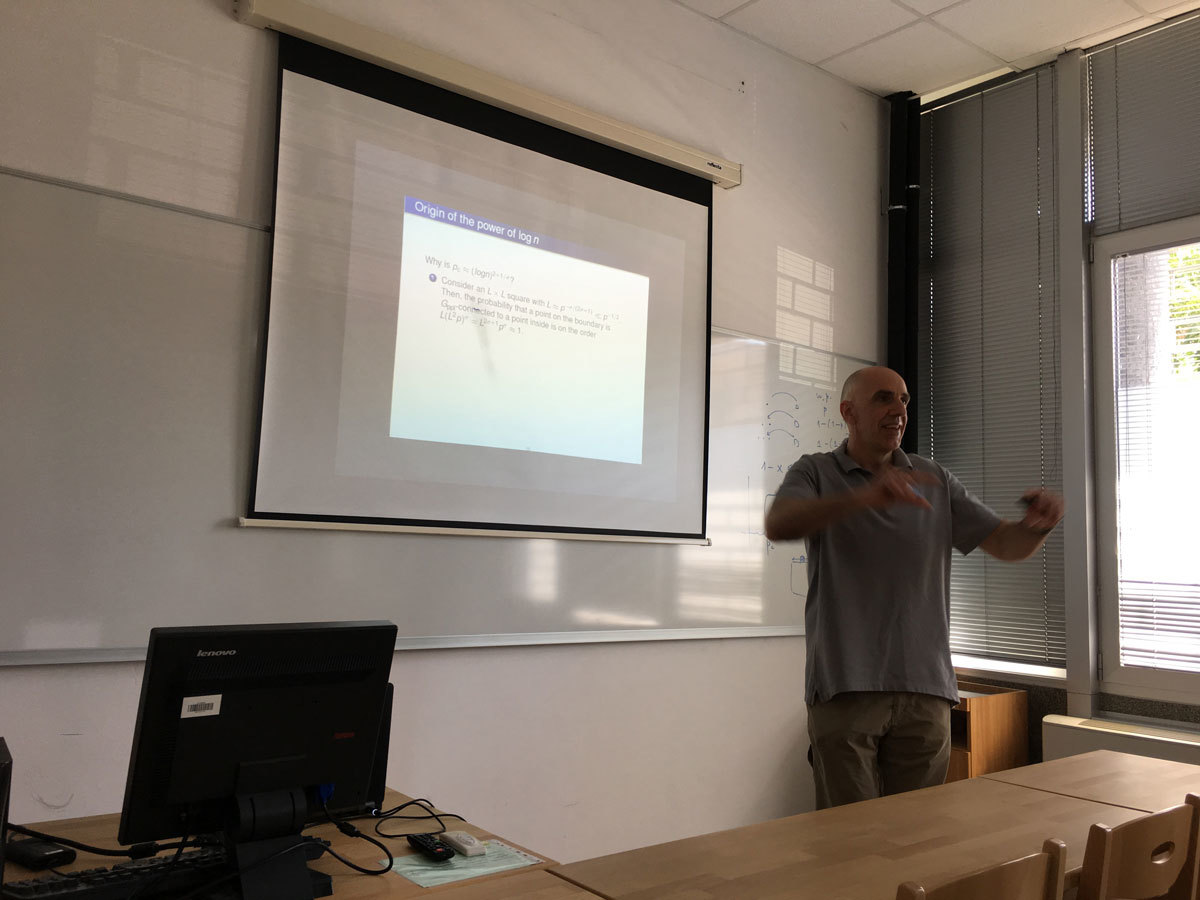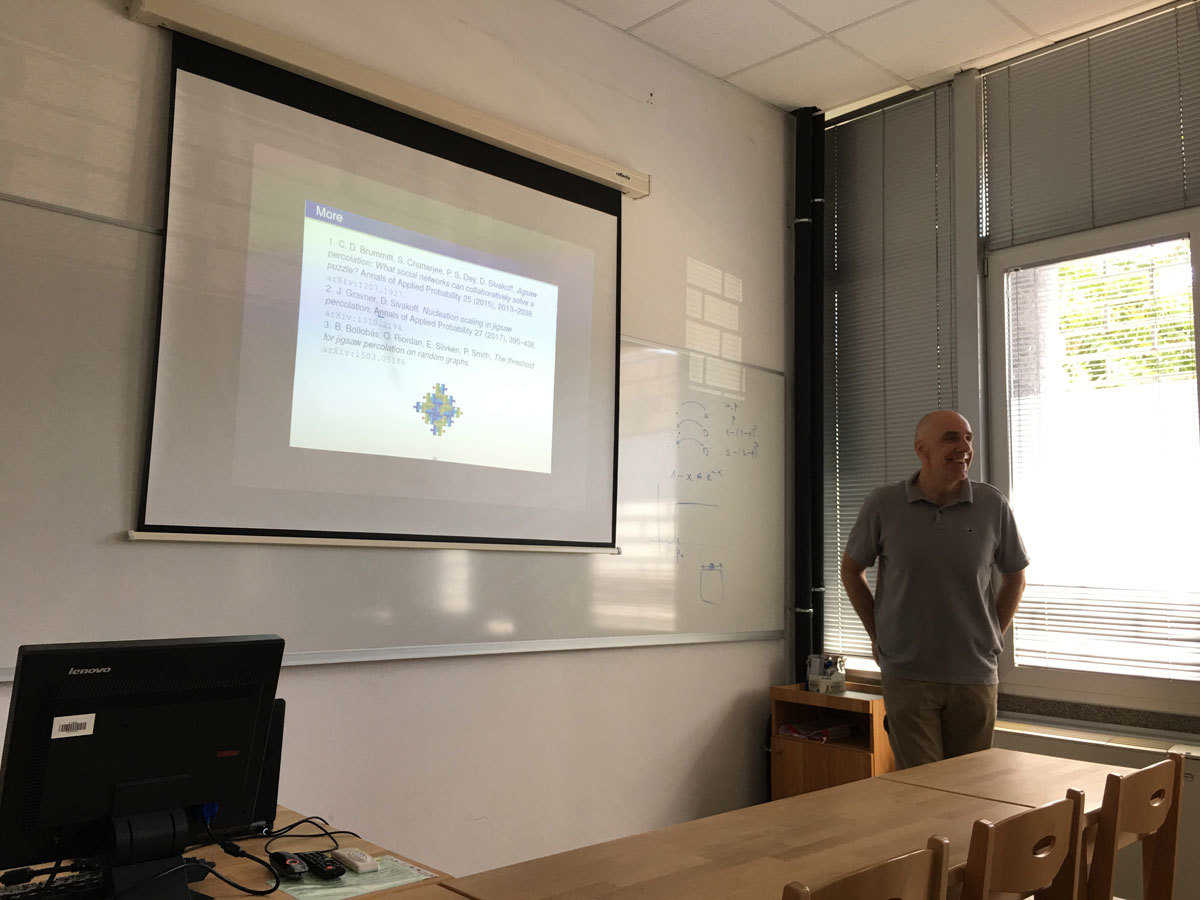Datum in ura / Date and time: 22.5.17
(10:00-11:00)
Predavalnica / Location: FAMNIT-POŠTA
Predavatelj / Lecturer: Jean-Florent Raymond (Faculty of Mathematics, Informatics and Mechanics of the University of Warsaw (Poland) and to LIRMM (France))
Naslov / Title: Polynomial expansion and sublinear balanced separators
Vsebina / Abstract:

The notion of bounded expansion has been introduced by Nešetřil and Ossona de Mendez as a general concept of sparsity. Classes of graphs of bounded expansion are interesting as they generalize many known sparse classes (as planar graphs, graphs of bounded degree, graphs excluding a minor, etc.). Moreover, several algorithmic problems that are known to be computationally hard in general turn out to be solvable in polynomial time when the inputs are taken in a class of bounded expansion fixed in advance.

On the other hand, classes of graphs with (balanced) sublinear separators are extensively used in the design of algorithms (with "Divide and Conquer" type techniques for instance) and enjoy nice structural properties. An example of graphs with sublinear separators is the class of planar graphs, by a classic result of Lipton and Tarjan.

The topic of this talk is the links between these two notions. Let C be a subgraph-closed class of graphs. Dvořák and Norin proved that graphs in C have sublinear balanced separators iff they have polynomial expansion. They conjectured that if the balanced separators in C have size O(n^{1−δ}) (for some 0 < δ ≤ 1), then the expansion in C is O(k^{c/δ}), for some constant c. We prove this conjecture. This is joint work with Louis Esperet.

Datum in ura / Date and time: 17.5.17
(13:00 - 14:00)
Predavalnica / Location: FAMNIT-MP1
Predavatelj / Lecturer: Safet Penjić (UP IAM)
Naslov / Title: On the Terwilliger Algebra of Bipartite Distance-regular graphs (Disposition of the PhD Thesis)
Vsebina / Abstract:

This is a presentation of the PhD thesis topic.

Let \G denote a bipartite distance-regular graph with diameter D \ge 4 and valency k \ge 3. Let X denote the vertex set of \G, and let A denote the adjacency matrix of \G. For x \in X and for 0 \le i \le D, let \G_i(x) denote the set of vertices in X that are distance i from vertex x. Define parameters \Delta_i (1\le i\le D-1) in terms of the intersection numbers by \Delta_i=(b_{i-1} - 1)(c_{i+1} - 1) - (c_2 - 1)p^i_{2i}. In PhD thesis we show that \Delta_2 = 0 implies D \le 5 or c_2 \in \{1,2\}.

For x \in X let T=T(x) denote the subalgebra of \MX generated by A, \Es_0, \Es_1, \ldots, \Es_D, where for 0 \le i \le D, \Es_i represents the projection onto the i-th subconstituent of \G with respect to x. We refer to T as the Terwilliger algebra of \G with respect to x. By the endpoint of an irreducible T-module W we mean \min\{i | \Es_iW \ne 0\}.

Consider a bipartite DRG \G with one of the following properties:

(b.1) \G has, up to isomorphism, a unique irreducible T-module W of endpoint 2, this module is not thin, \dim(\Es_iW)\le 2 for every i (2\le i\le D-1) and \dim(\Es_2W)= 1.

(b.2) \Delta_i=0 for every i (2\le i\le D-1).

(b.3) \G has the property that for 2\le i\le D-1, there exist complex scalars \alpha_i, \beta_i such that for all x,y,z\in X with \partial(x,y)=2, \partial(x,z)=i, \partial(y,z)=i, we have \alpha_i+\beta_i|\G_1(x)\cap\G_1(y)\cap\G_{i-1}(z)|=|\G_{i-1}(x)\cap\G_{i-1}(y)\cap\G_{1}(z)|.

(b.4) \Delta_2=0, \Delta_i\not=0 for at least one i (3\le i\le D-2), and (b.3) holds.

In this PhD Thesis we will show that the property (b.4) implies property (b.1). Note that property (b.4) includes (b.3) by definition, and we are interested in bipartite distance-regular graphs with property (b.3) because they arise as a natural family in the study of the Terwilliger algebra of a bipartite distance-regular graph, as we will see by the following  very important example. Suppose that \G is Q-polynomial. Then \G has, up to isomorphism, at most one irreducible T-module of endpoint 2 and diameter D-2, at most one irreducible T-module of endpoint 2 and diameter D-4 (they are both thin), and no other irreducible T-modules of endpoint 2, see [J. S. Caughman, IV, The Terwilliger algebras of bipartite P- and Q-polynomial schemes, Discrete Math. 196 (1999), 65--95.]. Furthermore, Terwilliger's {\it balanced set condition} ([P. Terwilliger, A new inequality for distance-regular graphs, Discrete Math. 137 (1995), 319--332.]) implies the property (b.3) ([Š. Miklavič, On bipartite Q-polynomial distance-regular graphs, European J. Combin. 28 (2007), 94--110.]).

In this PhD Thesis, we will not assume the Q-polynomial property for \G, but rather the property (b.3) above, together with \Delta_2=0. It is our goal to describe the irreducible T-modules with endpoint 2 for this case. We assume that \Delta_i\not=0 for at least one i (3\le i\le D-2), since graphs with property (b.2) are already well-understood ([B. Curtin, Almost 2-homogeneous bipartite distance-regular graphs, European J. Combin. 21 (2000), 865--876.]). First we will show that in case when c_2\le 2, there exists a certain equitable partition of the vertex set of \G which, for case c_2=1, involves 3(D-1)+\ell cells and, for case c_2=2, involves 4(D-1)+2\ell cells, for some integer \ell with 0\le\ell\le D-2. We use this equitable partition to describe the irreducible T-modules with endpoint 2 for this case.

In the second part of the thesis we assume \G is a bipartite Q-polynomial distance-regular graph with diameter D \ge 4, valency k \ge 3 and intersection numbers b_i, c_i. Caughman proved in [J. S. Caughman, IV, Bipartite Q-polynomial distance-regular graphs, Graphs Combin. 20 (2004), 47--57.] that if D \ge 12 then \G is either the D-dimensional hypercube, or the antipodal quotient of the 2D-dimensional hypercube, or the intersection numbers of \G satisfy c_i = (q^i-1)/(q-1) \; (0 \le i \le D) for some integer q at least 2. Note that if c_2 \le 2, then the last of the above possibilities cannot occur. The aim of this PhD thesis will also be to further investigate these graphs. We will show that if c_2 \le 2 then \G is either the D-dimensional hypercube, or the antipodal quotient of the 2D-dimensional hypercube, or D=5.

In the problems of (i) finding equitable partition for c_2\le 2; (ii) describing irreducible T-modules with endpoint 2 and (iii) proving that Q-polynomial distance-regular graph \G is either the D-dimensional hypercube, or the antipodal quotient of the 2D-dimensional hypercube, or D=5; we use combinatorial and linear algebra methods similar to those that are used in [Š. Miklavič and S. Penjić, "On bipartite Q-polynomial distance-regular graphs with c_2 ≤ 2", The Electronic Journal of Combinatorics 21(4) (2014), #P4.53.], [M. S. MacLeana, Š. Miklavič, S. Penjić, "On the Terwilliger algebra of bipartite distance-regular graphs with \Delta_2=0 and c_2=1", Linear Algebra and its Applications 496 (2016), 307–330.] and [S. Penjić, "On the Terwilliger algebra of bipartite distance-regular graphs with \Delta_2=0 and c_2=2", Discrete Mathematics 340 (2017), 452–466].

Datum in ura / Date and time: 15.5.17
(10:00-11:00)
Predavalnica / Location: FAMNIT-POŠTA
Predavatelj / Lecturer: Russ Woodroofe (Mississippi State University, USA)
Naslov / Title: Arrangements and the independence polynomial
Vsebina / Abstract:

I'll show how to construct a subspace arrangement which encodes the independence polynomial of a graph G.  I'll discuss possible applications to unimodality questions.

Datum in ura / Date and time: 8.5.17
(10:00-11:00)
Predavalnica / Location: FAMNIT-POŠTA
Predavatelj / Lecturer: Bogdan Soban
Naslov / Title: Matematika in digitalna umetnost
Vsebina / Abstract:

Povezanost med umetnostjo in znanostjo, še posebej z matematiko, je zgodovinsko dokazana. S pojavom digitalne umetnosti je matematika pridobila še dodatno vlogo, saj se neposredno vključuje v sam proces nastajanja umetniških del. Tudi preprost programski algoritem ne more brez pomoči matematike narisati niti najenostavnejših elementov slike. Moja raziskovanja na področju razvoja programske opreme za generiranje slik so bila zato vedno tesno povezana z iskanjem inovativnih rešitev v okviru poznanih matematičnih principov. V okviru predavanja bom predstavil osnovna izhodišča za risanje slike v ravnini s kartezičnimi in polarnimi koordinatami ter risanje slike v kompleksni ravnini. Poleg nazornega prikaza »vizualizacije« konkretnih matematičnih izrazov bom demonstriral delovanje parih programov, ki uporabljajo opisane koncepte.

e-mail: bogdan@soban-art.com
spletna stran: www.soban-art.com